# The Omnitruncated Tesseract

The omnitruncated tesseract is a uniform polychoron bounded by 80 cells (8 great rhombicuboctahedra, 16 truncated octahedra, 24 octagonal prisms, 32 hexagonal prisms), 464 polygons (288 squares, 128 hexagons, 48 octagons), 768 edges, and 384 vertices.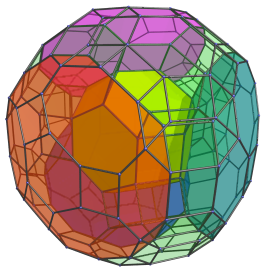The name “omnitruncated” means that it is thought of as a tesseract with each vertex, edge, face, and cell truncated. This is not precisely the case, since truncating the tesseract in this manner does not yield a uniform polychoron, but rather a non-uniform polychoron with the same topology as the omnitruncated tesseract.

Another, more precise way of constructing the omnitruncated tesseract is to place 8 equal great rhombicuboctahedra in the bounding hyperplanes of a tesseract and then expand them outwardly along each coordinate axis until the distance between the octagonal faces of two adjacent great rhombicuboctahedra is equal to the edge length. The remaining gaps can then be filled up with uniform octagonal prisms, hexagonal prisms, and truncated octahedra such that they enclose a 4D hypervolume. The result is the uniform omnitruncated tesseract.

## Structure

We shall explore the structure of the omnitruncated tesseract by means of its parallel projection into 3D, centered on one of the great rhombicuboctahedral cells.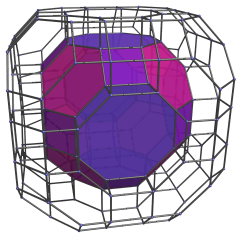The nearest cell, a great rhombicuboctahedron, projects to the center of this image. The octagonal faces of this cell are joined to 6 octagonal prisms, as shown in the next image: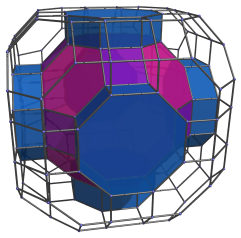The square faces of the nearest cell are joined to 12 hexagonal prisms, shown in the next image: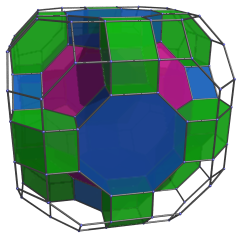The hexagonal faces of the nearest cell are joined to 8 truncated octahedra, which fit into the gaps visible in the above image. The next image shows these cells filled in: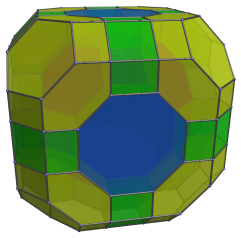We are now at the “equator” of the omnitruncated tesseract. There are 6 great rhombicuboctahedra at the equator, joined to the octagonal prisms we saw. The following image shows these cells: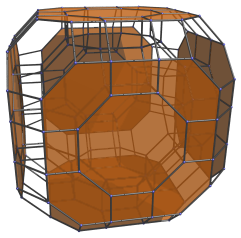For clarity, we've omitted the previous cells we have seen. The great rhombicuboctahedra have been foreshortened into non-regular octagons because they are orthogonal to the 4D viewpoint.

There are also 12 octagonal prisms at the equator, shown in the following image: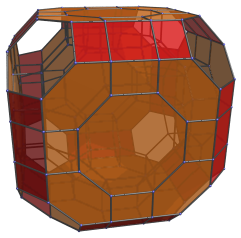These octagonal prisms lie at a 90° angle to the 4D viewpoint, so they have been foreshortened into rectangles.

Finally, there are 8 hexagonal prisms at the equator, shown in the next image: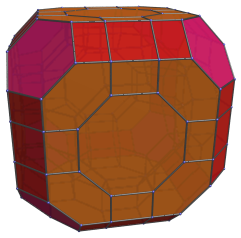Following these cells, we have cells on the far side of the omnitruncated tesseract, with a layout that exactly mirrors the layout of the first layers of cells we saw. This gives us a total of 1+6+1=8 great rhombicuboctahedra, 6+12+6=24 octagonal prisms, 12+8+12=32 hexagonal prisms, and 8+8=16 truncated octahedra.

The omnitruncated tesseract has a structure analogous to the great rhombicuboctahedron, with its great rhombicuboctahedral cells corresponding with the octagonal faces of the great rhombicuboctahedron, its truncated octahedra corresponding with the hexagonal faces, and its prisms corresponding with the square faces.

## Perspective projections

The following image shows a perspective projection of the omnitruncated tesseract into 3D, also centered on a great rhombicuboctahedron. It has the same layout of cells as we have seen in the parallel projection.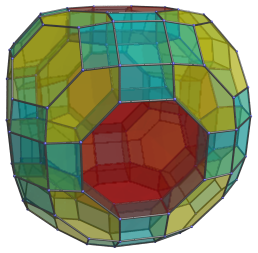We have colored each different type of face differently, rather than emphasize any particular cell.

The next image shows a different perspective projection of the omnitruncated tesseract, from another 4D viewpoint, this time centered on a truncated octahedron:The nearest truncated octahedron is shown in yellow, and the four surrounding great rhombicuboctahedra are colored in red, magenta, blue, and dark cyan. The great rhombicuboctahedra appear somewhat flattened because they are seen from an angle. For clarity, all the other cells are rendered in transparent green, and cells on the far side of the omnitruncated tesseract have been omitted.

## Coordinates

The coordinates of the vertices of the omnitruncated tesseract with edge length 2 are all permutations of coordinates and changes of sign of:

• (1, 1+√2, 1+2√2, 1+3√2)

Again we see the analogy with the great rhombicuboctahedron, whose coordinates are permutations of (1, 1+√2, 1+2√2).

Last updated 17 Jun 2019.